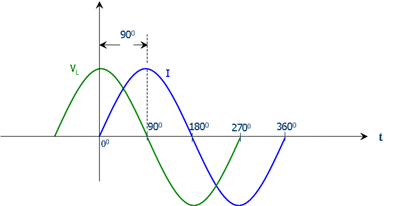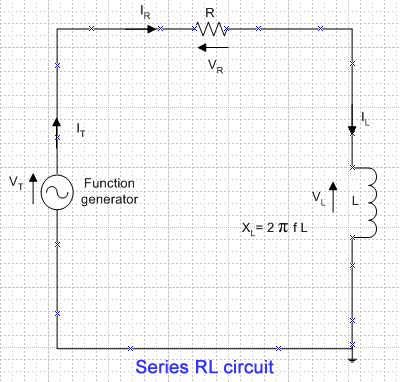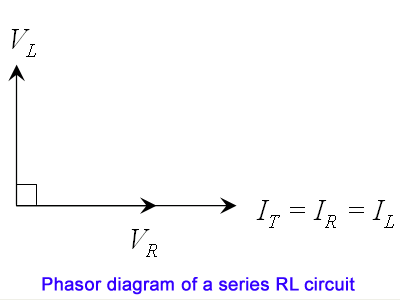### Series RL circuit (Rev: 1.3)Diagram 1: Inductor voltage (VL) and current (I) waveforms

In a pure inductor, current flows across the inductor lags the inductor voltage (VL) by 90 degree (diagram 1). In another words, inductor voltage (VL) leads the current by 90 degree (diagram 1). This is because when the voltage is applied to an inductor, the back ElectroMotive Force (EMF) [Lenz's law] resists current build up in the inductor, causing the inductor current (IL) to lag in time and phase than the inductor voltage (VL). The reactance of an inductor (XL) is the result of the inductor producing a back EMF which opposes change in the current.Diagram 2: Series RL circuit (assume pure inductor)

Digital Storage Oscilloscope (DSO) measures and displays AC and DC voltages signals over a wide range of frequencies. DSO does not measures current. When using the DSO to plot the inductor voltage (VL) and resistor voltage (VR) on a series RL circuit (diagram 2) with a constant current, we can conclude the relationship of the phase of current to the inductor voltage (VL) and resistor voltage (VR) by using the phasor diagram.

Phasor diagramDiagram 3: Phasor diagram of a series RL circuit (assume pure inductor)

According to Kirchoff's current law, the current at any given instant in a series circuit must be the same throughout all parts of the circuit. Therefore, total current (IT) = resistor current (IR) = inductor current (IL) as shown in the phasor diagram (diagram 3) of a series RL circuit (diagram 2).

Since current is the only quantity which is common to all parts of a series circuit, it is used as a reference for zero phase (positive x-axis) to show the phase relationships between currents and voltages.

Since the resistor current (IR) and resistor voltage (VR) are in phase (zero phase), the resistor voltage (VR) phasor will be on the same line on the positive x-axis. As the inductor voltage (VL) leads the current by 90 degree (assume pure inductor), the inductor voltage phase is a positive 90 degree.

References:

 Phase relationships in AC circuits

 Wikipedia

 Basic electricity and electronics (By Charles A. Schuler and Richard J. Fowler)Anonymous said...

what is the waveform of istantaneous power

Jack Ng said...

The input is an AC signal (sine wave at different frequencies) that is generated by a function generator.

## Please donate. Thanks.

paypal.me/jackngch
(PayPal-to-Paypal account only)